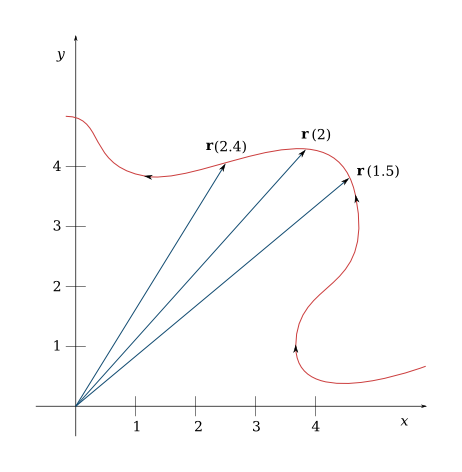Motion In Space

(requires JavaScript)

1. The following table gives coordinates of a particle moving through space along a smooth curve. Find the average velocities over the time intervals $\left[0,1\right]$, $\left[0.5,1\right]$, $\left[1,2\right]$, and $\left[1,1.5\right]$. Estimate the velocity and speed of the particle at $t=1$.

$t$$x$$y$$z$
$0.0$$2.7$$9.8$$3.7$
$0.5$$3.5$$7.2$$3.3$
$1.0$$4.5$$6.0$$3.0$
$1.5$$5.9$$6.4$$2.8$
$2.0$$7.3$$7.8$$2.7$

Average velocities are $⟨1.8,-3.8,-0.7⟩$, $⟨2,-2.4,-0.6⟩$, $⟨2.8,1.8,-0.3⟩$, and $⟨2.8,0.8,-0.4⟩$ respectively. When $t=1$, velocity is $2.4\mathbf{i}-0.8\mathbf{j}-0.5\mathbf{k}$ and speed is $2.58$.
2. The figure shows the path of a particle that moves with position vector $\mathbf{r}\left(t\right)$ at time $t$.1. Draw a vector that represents the average velocity of the particle over the time interval $2\le t\le 2.4$.
2. Draw a vector that represents the average velocity of the particle over the time interval $1.5\le t\le 2$
3. Write an expression for the velocity vector $\mathbf{v}\left(2\right)$.
4. Draw an approximation to the velocity vector $\mathbf{v}\left(2\right)$ and estimate the speed of the particle at $t=2$.
3. Find the velocity, acceleration, and speed of an electron with the position function

$\mathbf{r}\left(t\right)={e}^{t}\mathbf{i}+{e}^{2t}\mathbf{j}$

Sketch the path of the electron and draw the velocity and acceleration vectors for $t=0$.

$⟨{e}^{t},2{e}^{2t}⟩$, $⟨{e}^{t},4{e}^{2t}⟩$, ${e}^{t}\sqrt{1+4{e}^{2t}}$
4. Find the velocity, acceleration, and speed of an electron with the position function

$\mathbf{r}\left(t\right)=t\mathbf{i}+2\mathrm{cos}\left(t\right)\mathbf{j}+\mathrm{sin}\left(t\right)\mathbf{k}$

Sketch the path of the electron and draw the velocity and acceleration vectors for $t=0$.

$⟨1,-2\mathrm{sin}\left(t\right),\mathrm{cos}\left(t\right)⟩$, $⟨0,-2\mathrm{cos}\left(t\right),-\mathrm{sin}\left(t\right)⟩$, $\sqrt{2+3{\mathrm{sin}}^{2}\left(t\right)}$
5. The position function of a neutrino is given by

$\mathbf{r}\left(t\right)=⟨{t}^{2},5t,{t}^{2}-16t⟩$

When is the speed at minimum?

$\mathbf{v}\left(t\right)=⟨2t,5,2t-16⟩$,

so the speed is $\left|\mathbf{v}\left(t\right)\right|=\sqrt{8{t}^{2}-64t+281}$.

The function under the root sign is a parabola, and its minimal value is at the vertex $t=4$.

6. A ball is thrown at an angle of $45°$ to the horizontal ground. If the ball lands $90$ m away, what was the initial speed of the ball?
$30$ m/s.